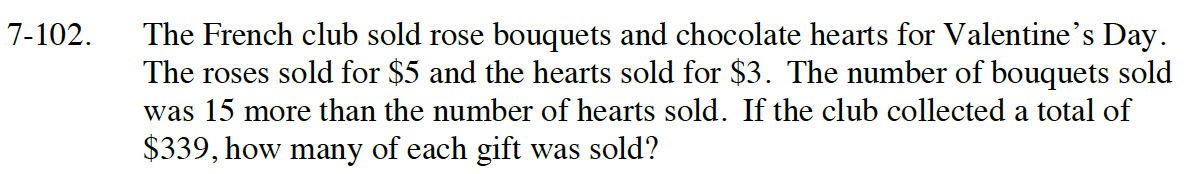Home > CC4 > Chapter 7 > Lesson 7.2.2 > Problem7-102

7-102.

The French club sold rose bouquets and chocolate hearts for Valentine’s Day. The roses sold for $5 and the hearts sold for$3. The number of bouquets sold was 15 more than the number of hearts sold. If the club collected a total of \$339, how many of each gift was sold? Homework Help ✎Think about using a system of equations, Let r = rose bouquets and c = chocolate hearts.

They sold 48 rose bouquets and 33 chocolate hearts.

Write both equations using r and c.
Equation #1: 5r + 3c =339,
Equation #2: r = c + 15.

Read Math Note box in section 6.2.3 on the Substitution Method. Use this method to solve these equations.